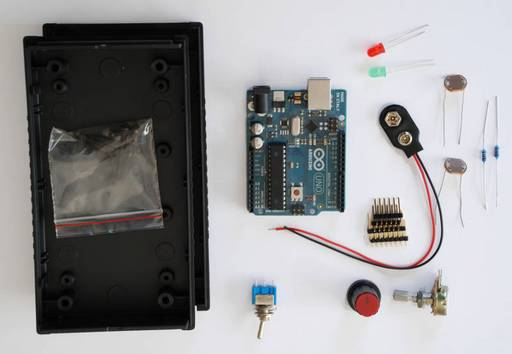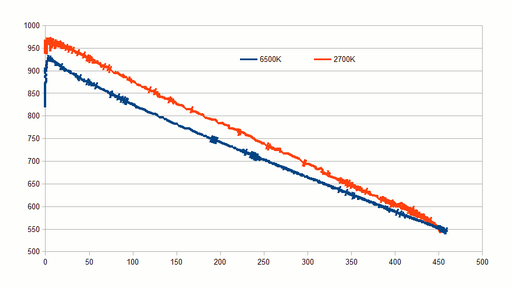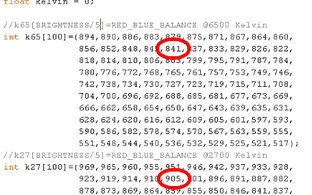DIY Color Temperature Meter

by viilaamo.com

Making of

In this example we use components that cost less than 40 euros just to demonstrate how it can be done.

Most expensive component is the Arduino board (22 euros) and the plastic housing (10 euros), the rest of the components cost total about 6 euros.

We used 10K resistors before and after the LDRs; +5V - 10K - LDR(Red) - LDR(Blue) - 10K - GND.One measurement point is between the LDRs and the other is between the blue LDR and 10K resistor. Smaller resistors give better accuracy on the bright end and larger resistors give better accuracy on the dark end. The measurement point between LDRs gives 2.5 volts (512 digital) when the light on both LDRs is equal, more than 2.5 volts when there is more red light and less than 2.5 volts when there is more blue light. The second measurement point gives 2.5 volts on very bright light (when the LDRs resistance is fraction of the 10K resistors) and zero volts on the dark (LDR resitance is mega ohms). The second measurepoint is needed for calibration purposes, since in practise the difference depends on the amount of light available (and the resistors selected).

After soldering everything together and making sure it works, calibration is needed at least with two different known color temperatures. We used 2700K with standard LED light bulb and 6500K cloudy day outdoor light (since 6500K bulbs are difficult to get). When doing the calibration you need to have a setup where you can gradually increase the amount of light and measure the input values throughout the brightess range. Repeat the measurements in all selected color temperatures. Good measurement results are surprisingly easy to get, you can just print the values from both input pins with Serial.print() and copy paste that to spreadsheet to verify the results.

Please notice that the values that you are getting are specific to your setup and will differ from the theoretical values. E.g. at 6500K light the voltage between the LDRs is hardly 2.5 volts on all brightness values (from the second measurement point).As you notice, in very dark (zero lux) and very bright the results are ambiguous. With different resistor selection you can focus on dark (bigger resistance) or bright (smaller resistance) better. There needs to be some resistance since you do not want to connect your +5V to ground and risk burning your Arduino.We put the measured values into an array as a function of brightness with steps of 5.

E.g. if the brightness sensor gives value 72 then we divide that with 5 (==14) and from relevant index positions we can see that the red-blue balance at 2700K is 905 and at 6500 is 841.

Now we can see the red-blue balance value and interpolate the correct color temperature. Quite straightforward as you can see.

The last thing to do is to map the color temperatures to values from the potentiometer (0-1023).

We indicate the position with two LEDs, red illuminated means you need to increase the temperature and green illuminated means that you have to de-crease the temperature.

Using the above example values, and linear interpolation from 2700K (905) to 6500K (841); if the red-blue balance value is 873 then we calculate the relevant potentiometer value by just scaling up (905-873)/(905-841)*1023 == 511. Which means color temperature half way between 2700K and 6500K (== 4600K) if using linear interpolation.

If the value from potentionmeter is below 511 then we illuminate the red LED and if the value is below 511 then we illuminate the green LED. If close enough to the center (+-10) then we illuminate the both.

//first turn off the LEDs
digitalWrite(ledPinRED, LOW);
digitalWrite(ledPinGREEN, LOW);

//if we are not in zero lux
if (brightnessValue)
{
//+-10 illuminate both - we are close enough
if (potentiometerValue+10>((int) estimate))
{
digitalWrite(ledPinRED, HIGH);
}
if (potentiometerValue-10<((int) estimate))
{
digitalWrite(ledPinGREEN, HIGH);
}

You still need to print out measurement scale for the potentiometer and that's about it - the color meter is ready to be used.

Oh, yes, here is the code!